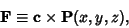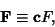## Vector Integral

The following vector integrals are related to the Curl Theorem. If(1)

then(2)

If(3)

then(4)

The following are related to the Divergence Theorem. If(5)

then(6)

Finally, if(7)

then(8)

See also Curl Theorem, Divergence Theorem, Gradient Theorem, Green's Identities, Line Integral, Surface Integral, Vector Derivative, Volume Integral